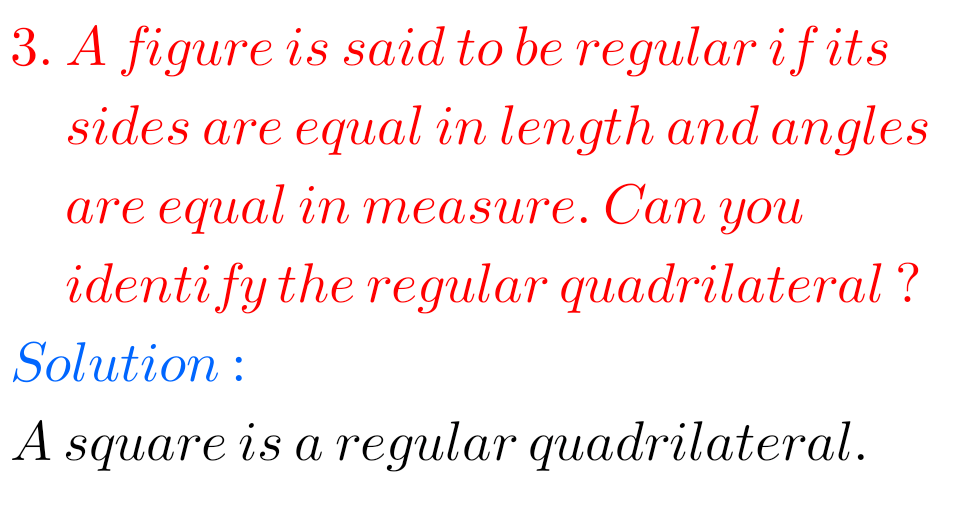## Maths solutions for exercise 5.7 chapter 6 class 6 Maths Ncert

Chapter 5 exercise 5.7 solutions 6th class maths NCERT NCERT Maths Class 6 chapter 5 Understanding Elementary Shapes Exercise 5.7 solutions are given. You should study the textbook lesson Understanding Elementary Shapes very well. You should observe the example problems and solutions given in the textbook. Observe the given solutions and try them in your …